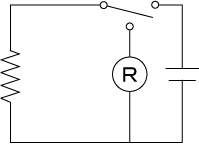### Resistance Method

March 28, 2020

The method for measuring a coil temperature rise:
Since the value of a coil temperature rise is easily effected by ambient temperature, measuring temperature on the surface of a coil cannot yield any accurate measurements. Thus, the mean temperature is determined by the resistance method using the temperature coefficient of resistance of copper.### Formula

T2 = (R2/R1 - 1) x (234.5 ＋ T1) + ΔT

R1: initial resistance
R2: final resistance after energization
T1: initial ambient temperature
T2: coil temperature rise
ΔT: change in ambient temperature from the beginning to the termination of energization
When the ambient temperature rises, ΔT is subtracted. When the ambient temperature falls, ΔT is added.